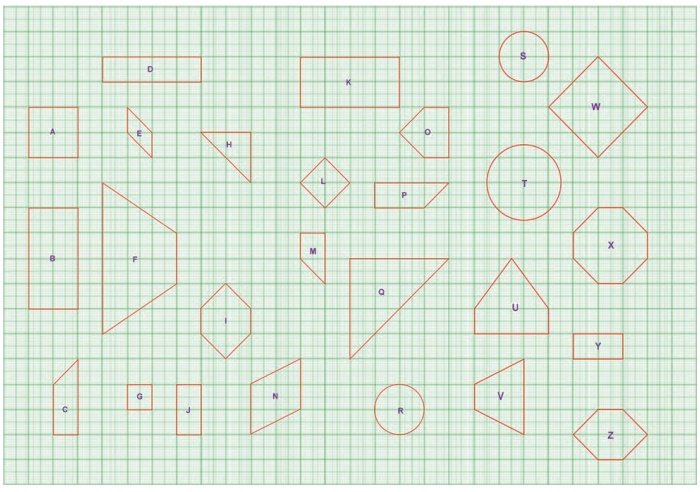Home | | Maths 8th Std | Congruent and Similar Shapes

# Congruent and Similar Shapes

Congruent figures are exactly the same in shape and size. In other words, shapes are congruent if one fits exactly over the other.

Congruent and Similar Shapes

Congruent figures are exactly the same in shape and size. In other words, shapes are congruent if one fits exactly over the other.

ExamplesSimilar figures mathematically have the same shape but different sizes. Two geometrical figures are said to be similar (~) if the measures of one to the corresponding measures of the other are in a constant ratio. In other words, every part of a photographic enlargement is similar to the corresponding part of the original.

ExamplesTry these

Identify the pairs of figures which are similar and congruent and write the letter pairs.Solution:

Similar shapes:

(i) W and L

(ii) B and J

(iii) A and G

(iv) B and J

(v) B and Y

(vi) E and N

(vii) H and Q

(viii) R and T

(ix) S and T

Congruent shapes:

(i) Z and I

(ii) J and Y

(iii) C and P

(iv) B and K

(v) R and S

(vi) I and Z

You can find more.

Tags : Geometry | Chapter 5 | 8th Maths , 8th Maths : Chapter 5 : Geometry
Study Material, Lecturing Notes, Assignment, Reference, Wiki description explanation, brief detail
8th Maths : Chapter 5 : Geometry : Congruent and Similar Shapes | Geometry | Chapter 5 | 8th Maths